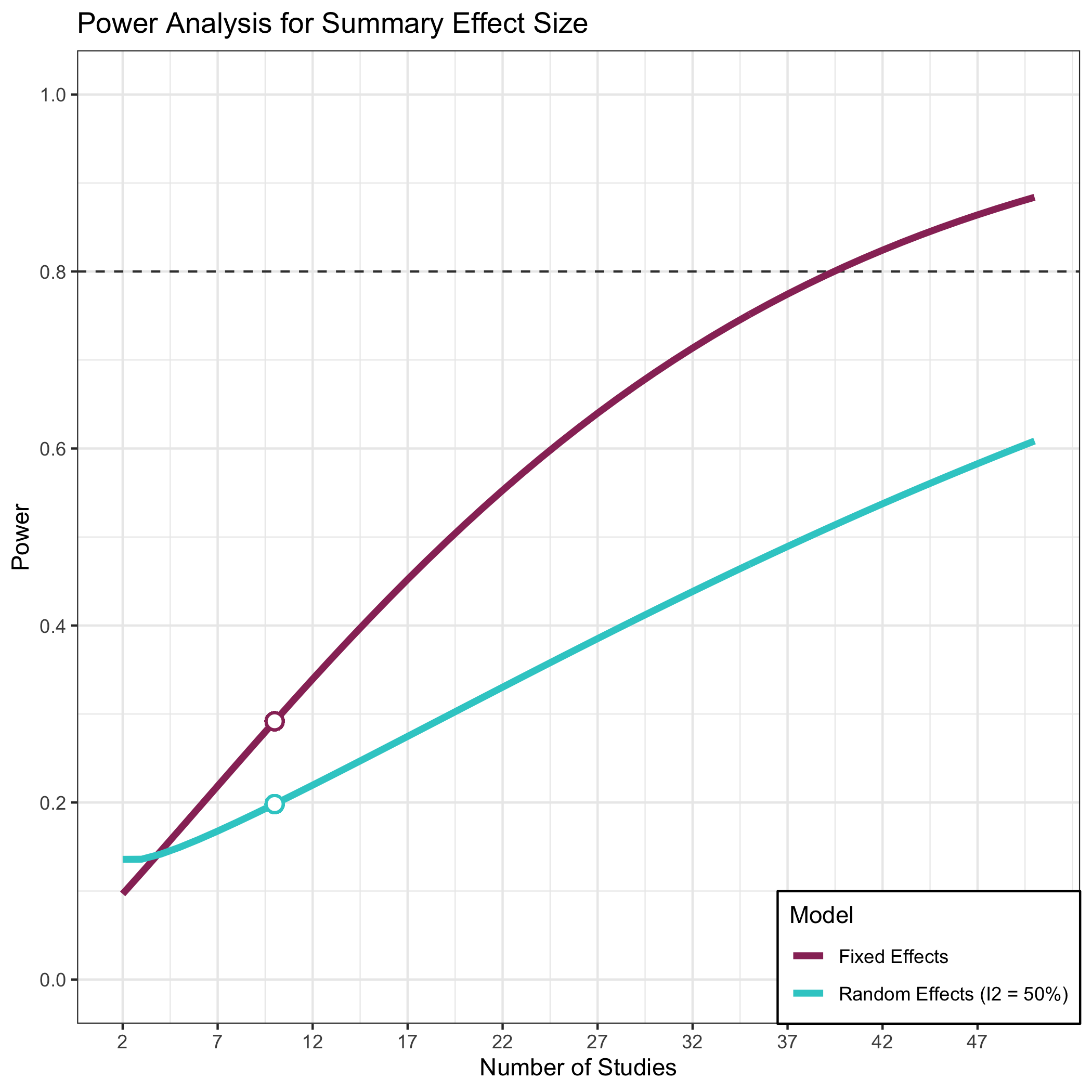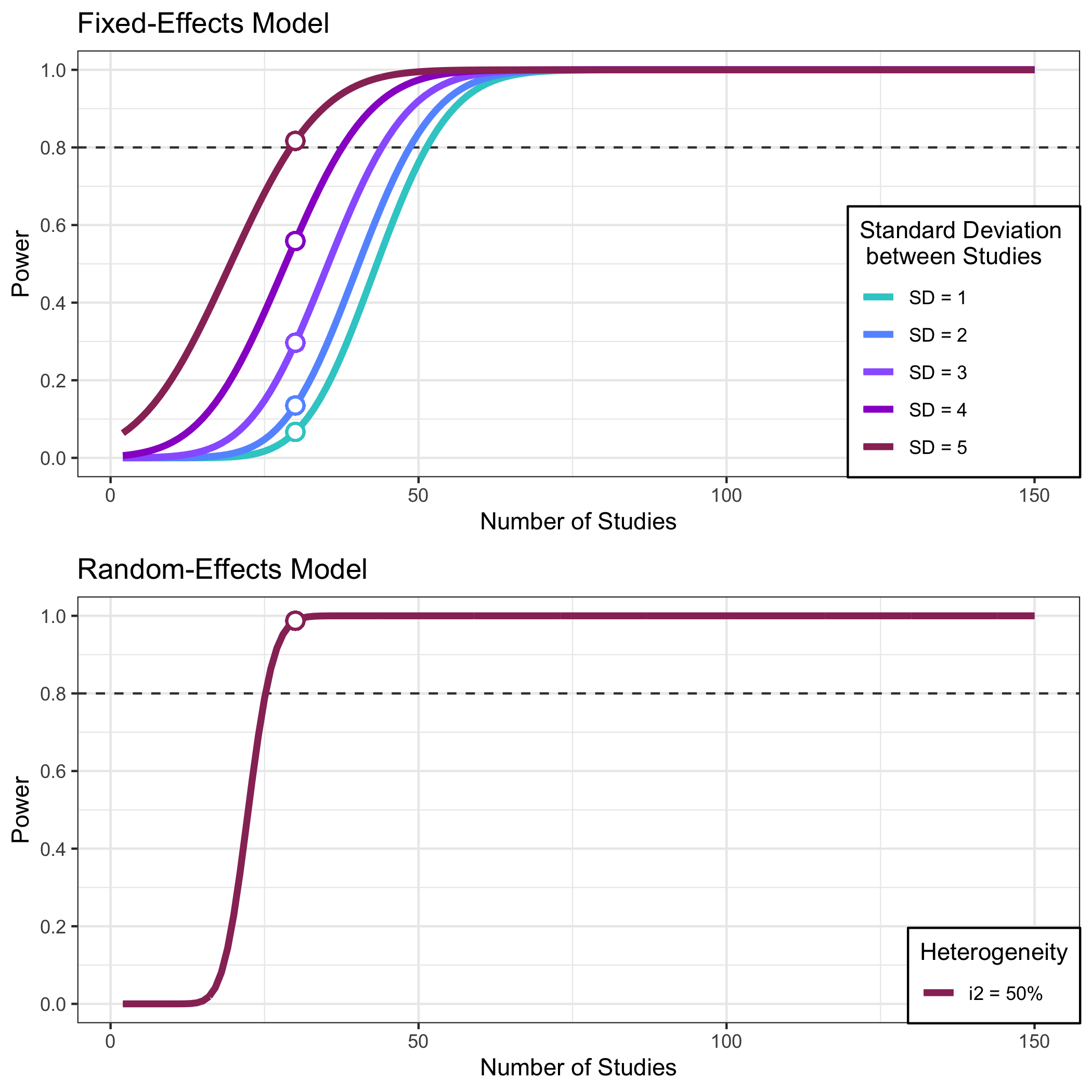Using-metapower

library(metapower)

Computing Meta-analytic Power

Suppose that we plan to meta-analyze all published findings to compute a summary effect size estimate for the group difference between typically developing individuals and individuals with autism on a measure of face recognition ability. In order to plan the study accordingly, we must choose plausible values for the following:

1. Expected effect size
2. Expected sample size per group
3. Expected number of studies
4. Expected Degree of heterogeneity (i.e., $$I^2$$)

for our meta-analysis of face recognition deficits in autism

1. We expect that face recognition deficits in ASD are small (Cohen’s d = 0.2)
2. Sample sizes in autism research are generally small. We expect the average group size to be 20.
3. Face recognition is frequently studied in autism; therefore, we expect to find 10 studies.
4. We anticipate moderate heterogeneity, $$I^2$$ = .50

To do this with metapower, we use the core function mpower()

my_power <- mpower(effect_size = .2, study_size = 20, k = 10, i2 = .50, es_type = "d")
print(my_power)
#>
#>  Power Analysis for Meta-analysis
#>
#>  Effect Size Metric:                d
#>  Expected Effect Size:              0.2
#>  Expected Study Size:               20
#>  Expected Number of Studies:        10
#>
#>  Estimated Power: Mean Effect Size
#>
#>  Fixed-Effects Model                0.2917841
#>  Random-Effects Model (i2 = 50%):   0.1982394

The first part of the output shows the expected input values, where the main results are shown in the bottom portion, mainly, Estimated Power. Under this set of values, our power to detect a mean difference under a Fixed-Effects model is 29.18%. Furthermore, we can look at the power under a Random-Effects model.

Given that power analysis require a lot of assumptions, it is generally advisable to look at power across a range of input values. To visualize the power curve for these set of input parameters, use plot_mpower() to generate a ggobject that is modifiable and by default, shows 5x as many studies as the user inputs.

plot_mpower(my_power)For fixed-effects model, power curves are shown for a range of effect sizes, whereas random-effects model shows power across a range of heterogeneity values, $$\tau^2$$

For users wanting more flexibility in visualization, the mpower object contains a data frame $power_range containing all data populating the ggobject, str(my_power$power_range)
#> 'data.frame':    147 obs. of  9 variables:
#>  $k_v : int 2 3 4 5 6 7 8 9 10 11 ... #>$ es_v        : num  0.2 0.2 0.2 0.2 0.2 0.2 0.2 0.2 0.2 0.2 ...
#>  $n_v : num 20 20 20 20 20 20 20 20 20 20 ... #>$ i2          : num  0.5 0.5 0.5 0.5 0.5 0.5 0.5 0.5 0.5 0.5 ...
#>  $c_alpha : num 1.96 1.96 1.96 1.96 1.96 ... #>$ test_type   : chr  "two-tailed" "two-tailed" "two-tailed" "two-tailed" ...
#>  $variance : num 0.201 0.201 0.201 0.201 0.201 0.201 0.201 0.201 0.201 0.201 ... #>$ fixed_power : num  0.0967 0.1207 0.145 0.1695 0.194 ...
#>  \$ random_power: num  0.136 0.136 0.142 0.149 0.158 ...

Power for the Test of Homogeneity

For Fixed-Effects Model, the test of homogeneity examines whether the amount of variation among effect sizes is greater than that of sampling error alone. To compute this, simply wrap the same arguments used in mpower with homogen_power. Since it is hard to justify selecting the standard deviation between a set of studies, homogen_power() automatically computes power across a range of different standard deviation values (i.e., SD = 1, SD = 2.)

(my_homogen_power <- homogen_power(effect_size = .25, study_size = 20, k = 30,i2 = .50, es_type = "d"))
#>
#>  Power Analysis for Test of Homogeneity in Meta-analysis
#>
#>  Effect Size Metric:                d
#>  Expected Effect Size:              0.25
#>  Expected Study Size:               20
#>  Expected Number of Studies:        30
#>
#>  Estimated Power: Test of Homogeneity
#>
#>  Fixed-Effects Model (SD = 1)        0.06687224
#>  Fixed-Effects Model (SD = 2)        0.1348743
#>  Fixed-Effects Model (SD = 3)        0.2966788
#>  Fixed-Effects Model (SD = 4)        0.55878
#>  Fixed-Effects Model (SD = 5)        0.8167712
#>
#>  Random-Effects Model (i2 = 50%):    0.9872307

For Random-Effects models, homogen_power() computes power given the user-specified heterogeneity value (i.e., $$I^2$$)

In this example, we specified i2 = .50, but below are common benchmarks:

1. $$I^2$$ = 0% (No Heterogeneity)
2. $$I^2$$ = 25% (Low Heterogeneity)
3. $$I^2$$ = 50% (Moderate Heterogeneity)
4. $$I^2$$ = 75% (Large Heterogeneity)

We can visualize power across a range with plot_homogen_power

plot_homogen_power(my_homogen_power)Example 2: Power analysis for moderation analysis (categorical variables)

Although researchers are primarily interested in conducting meta-analysis to quantify the main effect of a specific phenomenon, It is very common to evaluate the moderation of this overall effect based on a number of study- and/or sample-related characteristics such as task paradigm or age group (e.g., children, adolescents, adults). To compute the statistical power for the detection of categorical moderators, we use the function subgroup_power() with a few additional arguments, mainly:

1. Expected number of groups (n_groups):
2. Expected effect size of each group(effect_sizes):

for our meta-analysis of face recognition deficits in autism

We may expect that face recognition tasks have larger effect sizes then face perception tasks; therefore, we specify 2 groups and their respective expected effect sizes:

1. n_groups = 2
2. effect_sizes = c(.2,.5)
my_subgroup_power <- subgroup_power(n_groups = 2,
effect_sizes = c(.2,.5),
study_size = 20,
k = 30,
i2 = .5,
es_type = "d")
print(my_subgroup_power)
#>
#>  Power Analysis for Subgroup analysis:
#>
#>  Effect Size Metric:                d
#>  Number of Subgroups:               2
#>  Groups:
#>  Expected Effect Sizes:             0.2 0.5
#>  Expected Study Size:               20
#>  Expected Number of Studies:        30
#>
#>  Esimated Power to detect subgroup differences
#>
#>  Fixed-Effects Model:               0.4456862
#>  Random-Effects Model (i2 = 50%):   0.2517633
plot_subgroup_power(my_subgroup_power)